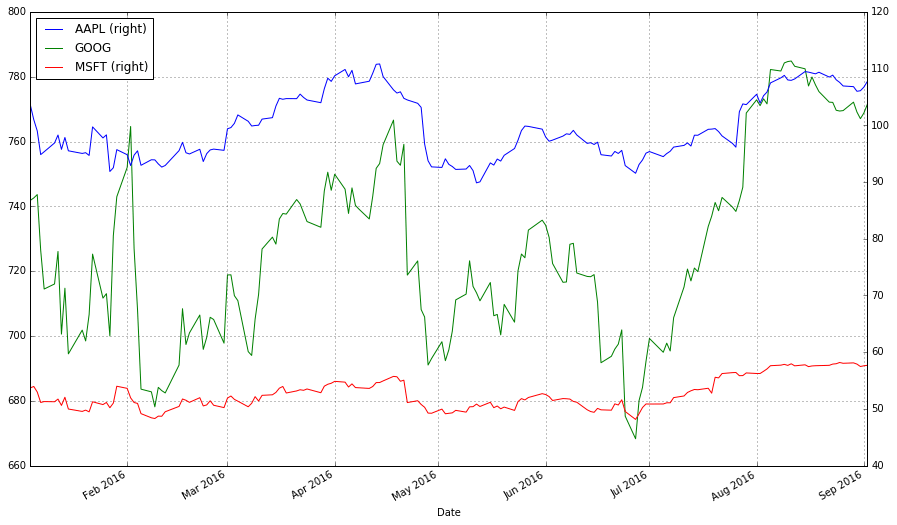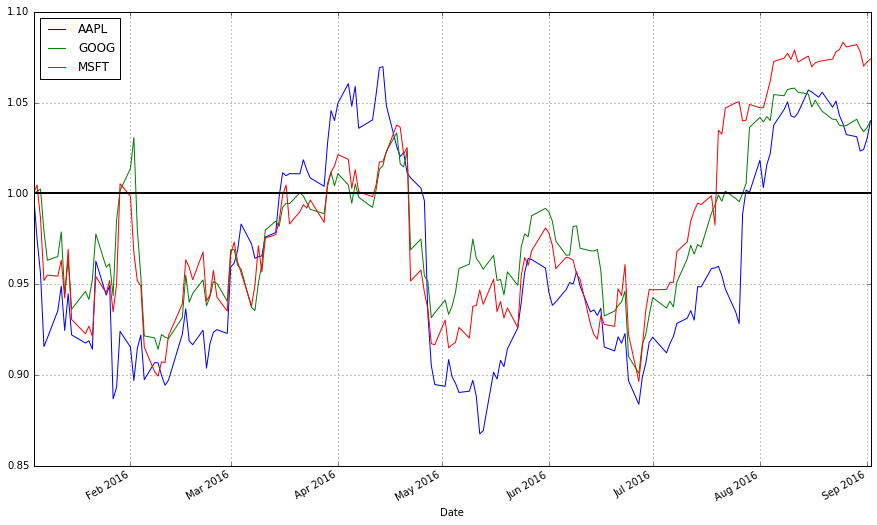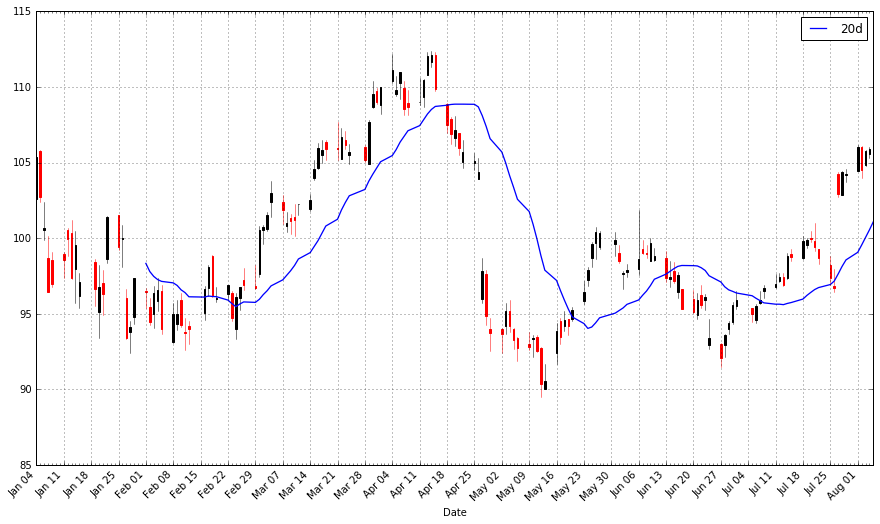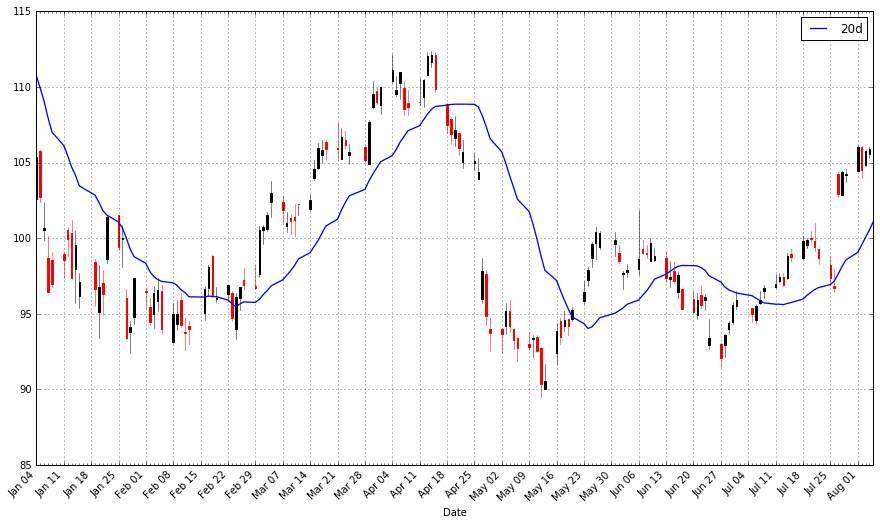# An Introduction to Stock Market Data Analysis with Python (Part 1)

This post is the first in a two-part series on stock data analysis using Python, based on a lecture I gave on the subject for MATH 3900 (Data Science) at the University of Utah. In these posts, I will discuss basics such as obtaining the data from Yahoo! Finance using pandas, visualizing stock data, moving averages, developing a moving-average crossover strategy, backtesting, and benchmarking. The final post will include practice problems. This first post discusses topics up to introducing moving averages.

NOTE: The information in this post is of a general nature containing information and opinions from the author’s perspective. None of the content of this post should be considered financial advice. Furthermore, any code written here is provided without any form of guarantee. Individuals who choose to use it do so at their own risk.

## Introduction

Advanced mathematics and statistics has been present in finance for some time. Prior to the 1980s, banking and finance were well known for being “boring”; investment banking was distinct from commercial banking and the primary role of the industry was handling “simple” (at least in comparison to today) financial instruments, such as loans. Deregulation under the Reagan administration, coupled with an influx of mathematical talent, transformed the industry from the “boring” business of banking to what it is today, and since then, finance has joined the other sciences as a motivation for mathematical research and advancement. For example one of the biggest recent achievements of mathematics was the derivation of the Black-Scholes formula, which facilitated the pricing of stock options (a contract giving the holder the right to purchase or sell a stock at a particular price to the issuer of the option). That said, bad statistical models, including the Black-Scholes formula, hold part of the blame for the 2008 financial crisis.

In recent years, computer science has joined advanced mathematics in revolutionizing finance and trading, the practice of buying and selling of financial assets for the purpose of making a profit. In recent years, trading has become dominated by computers; algorithms are responsible for making rapid split-second trading decisions faster than humans could make (so rapidly, the speed at which light travels is a limitation when designing systems). Additionally, machine learning and data mining techniques are growing in popularity in the financial sector, and likely will continue to do so. In fact, a large part of algorithmic trading is high-frequency trading (HFT). While algorithms may outperform humans, the technology is still new and playing in a famously turbulent, high-stakes arena. HFT was responsible for phenomena such as the 2010 flash crash and a 2013 flash crash prompted by a hacked Associated Press tweet about an attack on the White House.

This lecture, however, will not be about how to crash the stock market with bad mathematical models or trading algorithms. Instead, I intend to provide you with basic tools for handling and analyzing stock market data with Python. I will also discuss moving averages, how to construct trading strategies using moving averages, how to formulate exit strategies upon entering a position, and how to evaluate a strategy with backtesting.

DISCLAIMER: THIS IS NOT FINANCIAL ADVICE!!! Furthermore, I have ZERO experience as a trader (a lot of this knowledge comes from a one-semester course on stock trading I took at Salt Lake Community College)! This is purely introductory knowledge, not enough to make a living trading stocks. People can and do lose money trading stocks, and you do so at your own risk!

## Getting and Visualizing Stock Data

### Getting Data from Yahoo! Finance with pandas

Before we play with stock data, we need to get it in some workable format. Stock data can be obtained from Yahoo! Finance, Google Finance, or a number of other sources, and the pandas package provides easy access to Yahoo! Finance and Google Finance data, along with other sources. In this lecture, we will get our data from Yahoo! Finance.

The following code demonstrates how to create directly a DataFrame object containing stock information. (You can read more about remote data access here.)

 1 2 3 4 5 6 7 8 9 10 11 12 13 import pandas as pd import pandas.io.data as web   # Package and modules for importing data; this code may change depending on pandas version import datetime   # We will look at stock prices over the past year, starting at January 1, 2016 start = datetime.datetime(2016,1,1) end = datetime.date.today()   # Let’s get Apple stock data; Apple’s ticker symbol is AAPL # First argument is the series we want, second is the source (“yahoo” for Yahoo! Finance), third is the start date, fourth is the end date apple = web.DataReader(“AAPL”, “yahoo”, start, end)   type(apple)

Open High Low Close Volume Adj Close
Date
2016-01-04 102.610001 105.370003 102.000000 105.349998 67649400 103.586180
2016-01-05 105.750000 105.849998 102.410004 102.709999 55791000 100.990380
2016-01-06 100.559998 102.370003 99.870003 100.699997 68457400 99.014030
2016-01-07 98.680000 100.129997 96.430000 96.449997 81094400 94.835186
2016-01-08 98.550003 99.110001 96.760002 96.959999 70798000 95.336649

Let’s briefly discuss this. Open is the price of the stock at the beginning of the trading day (it need not be the closing price of the previous trading day), high is the highest price of the stock on that trading day, low the lowest price of the stock on that trading day, and close the price of the stock at closing time. Volume indicates how many stocks were traded. Adjusted close is the closing price of the stock that adjusts the price of the stock for corporate actions. While stock prices are considered to be set mostly by traders, stock splits (when the company makes each extant stock worth two and halves the price) and dividends (payout of company profits per share) also affect the price of a stock and should be accounted for.

### Visualizing Stock Data

Now that we have stock data we would like to visualize it. I first demonstrate how to do so using the matplotlib package. Notice that the apple DataFrame object has a convenience method, plot(), which makes creating plots easier.

 1 2 3 4 5 6 7 8 import matplotlib.pyplot as plt   # Import matplotlib # This line is necessary for the plot to appear in a Jupyter notebook %matplotlib inline # Control the default size of figures in this Jupyter notebook %pylab inline pylab.rcParams[‘figure.figsize’] = (15, 9)   # Change the size of plots   apple[“Adj Close”].plot(grid = True) # Plot the adjusted closing price of AAPLA linechart is fine, but there are at least four variables involved for each date (open, high, low, and close), and we would like to have some visual way to see all four variables that does not require plotting four separate lines. Financial data is often plotted with a Japanese candlestick plot, so named because it was first created by 18th century Japanese rice traders. Such a chart can be created with matplotlib, though it requires considerable effort.

I have made a function you are welcome to use to more easily create candlestick charts from pandas data frames, and use it to plot our stock data. (Code is based off this example, and you can read the documentation for the functions involved here.)

 1 2 3 4 5 6 7 8 9 10 11 12 13 14 15 16 17 18 19 20 21 22 23 24 25 26 27 28 29 30 31 32 33 34 35 36 37 38 39 40 41 42 43 44 45 46 47 48 49 50 51 52 53 54 55 56 57 58 59 60 61 62 63 64 65 66 67 68 69 70 71 72 73 74 75 76 77 78 79 80 81 82 83 84 85 86 from matplotlib.dates import DateFormatter, WeekdayLocator,\     DayLocator, MONDAY from matplotlib.finance import candlestick_ohlc   def pandas_candlestick_ohlc(dat, stick = “day”, otherseries = None):     “””     :param dat: pandas DataFrame object with datetime64 index, and float columns “Open”, “High”, “Low”, and “Close”, likely created via DataReader from “yahoo”     :param stick: A string or number indicating the period of time covered by a single candlestick. Valid string inputs include “day”, “week”, “month”, and “year”, (“day” default), and any numeric input indicates the number of trading days included in a period     :param otherseries: An iterable that will be coerced into a list, containing the columns of dat that hold other series to be plotted as lines       This will show a Japanese candlestick plot for stock data stored in dat, also plotting other series if passed.     “””     mondays = WeekdayLocator(MONDAY)        # major ticks on the mondays     alldays = DayLocator()              # minor ticks on the days     dayFormatter = DateFormatter(‘%d’)      # e.g., 12       # Create a new DataFrame which includes OHLC data for each period specified by stick input     transdat = dat.loc[:,[“Open”, “High”, “Low”, “Close”]]     if (type(stick) == str):         if stick == “day”:             plotdat = transdat             stick = 1 # Used for plotting         elif stick in [“week”, “month”, “year”]:             if stick == “week”:                 transdat[“week”] = pd.to_datetime(transdat.index).map(lambda x: x.isocalendar()) # Identify weeks             elif stick == “month”:                 transdat[“month”] = pd.to_datetime(transdat.index).map(lambda x: x.month) # Identify months             transdat[“year”] = pd.to_datetime(transdat.index).map(lambda x: x.isocalendar()) # Identify years             grouped = transdat.groupby(list(set([“year”,stick]))) # Group by year and other appropriate variable             plotdat = pd.DataFrame({“Open”: [], “High”: [], “Low”: [], “Close”: []}) # Create empty data frame containing what will be plotted             for name, group in grouped:                 plotdat = plotdat.append(pd.DataFrame({“Open”: group.iloc[0,0],                                             “High”: max(group.High),                                             “Low”: min(group.Low),                                             “Close”: group.iloc[-1,3]},                                            index = [group.index]))             if stick == “week”: stick = 5             elif stick == “month”: stick = 30             elif stick == “year”: stick = 365       elif (type(stick) == int and stick >= 1):         transdat[“stick”] = [np.floor(i / stick) for i in range(len(transdat.index))]         grouped = transdat.groupby(“stick”)         plotdat = pd.DataFrame({“Open”: [], “High”: [], “Low”: [], “Close”: []}) # Create empty data frame containing what will be plotted         for name, group in grouped:             plotdat = plotdat.append(pd.DataFrame({“Open”: group.iloc[0,0],                                         “High”: max(group.High),                                         “Low”: min(group.Low),                                         “Close”: group.iloc[-1,3]},                                        index = [group.index]))       else:         raise ValueError(‘Valid inputs to argument “stick” include the strings “day”, “week”, “month”, “year”, or a positive integer’)         # Set plot parameters, including the axis object ax used for plotting     fig, ax = plt.subplots()     fig.subplots_adjust(bottom=0.2)     if plotdat.index[-1] – plotdat.index < pd.Timedelta(‘730 days’):         weekFormatter = DateFormatter(‘%b %d’)  # e.g., Jan 12         ax.xaxis.set_major_locator(mondays)         ax.xaxis.set_minor_locator(alldays)     else:         weekFormatter = DateFormatter(‘%b %d, %Y’)     ax.xaxis.set_major_formatter(weekFormatter)       ax.grid(True)       # Create the candelstick chart     candlestick_ohlc(ax, list(zip(list(date2num(plotdat.index.tolist())), plotdat[“Open”].tolist(), plotdat[“High”].tolist(),                       plotdat[“Low”].tolist(), plotdat[“Close”].tolist())),                       colorup = “black”, colordown = “red”, width = stick * .4)       # Plot other series (such as moving averages) as lines     if otherseries != None:         if type(otherseries) != list:             otherseries = [otherseries]         dat.loc[:,otherseries].plot(ax = ax, lw = 1.3, grid = True)       ax.xaxis_date()     ax.autoscale_view()     plt.setp(plt.gca().get_xticklabels(), rotation=45, horizontalalignment=’right’)       plt.show()   pandas_candlestick_ohlc(apple)With a candlestick chart, a black candlestick indicates a day where the closing price was higher than the open (a gain), while a red candlestick indicates a day where the open was higher than the close (a loss). The wicks indicate the high and the low, and the body the open and close (hue is used to determine which end of the body is the open and which the close). Candlestick charts are popular in finance and some strategies in technical analysis use them to make trading decisions, depending on the shape, color, and position of the candles. I will not cover such strategies today.

We may wish to plot multiple financial instruments together; we may want to compare stocks, compare them to the market, or look at other securities such as exchange-traded funds (ETFs). Later, we will also want to see how to plot a financial instrument against some indicator, like a moving average. For this you would rather use a line chart than a candlestick chart. (How would you plot multiple candlestick charts on top of one another without cluttering the chart?)

Below, I get stock data for some other tech companies and plot their adjusted close together.

 1 2 3 4 5 6 7 8 9 microsoft = web.DataReader(“MSFT”, “yahoo”, start, end) google = web.DataReader(“GOOG”, “yahoo”, start, end)   # Below I create a DataFrame consisting of the adjusted closing price of these stocks, first by making a list of these objects and using the join method stocks = pd.DataFrame({“AAPL”: apple[“Adj Close”],                       “MSFT”: microsoft[“Adj Close”],                       “GOOG”: google[“Adj Close”]})   stocks.head()
AAPL GOOG MSFT
Date
2016-01-04 103.586180 741.840027 53.696756
2016-01-05 100.990380 742.580017 53.941723
2016-01-06 99.014030 743.619995 52.961855
2016-01-07 94.835186 726.390015 51.119702
2016-01-08 95.336649 714.469971 51.276485
 1 stocks.plot(grid = True)What’s wrong with this chart? While absolute price is important (pricy stocks are difficult to purchase, which affects not only their volatility but your ability to trade that stock), when trading, we are more concerned about the relative change of an asset rather than its absolute price. Google’s stocks are much more expensive than Apple’s or Microsoft’s, and this difference makes Apple’s and Microsoft’s stocks appear much less volatile than they truly are.

One solution would be to use two different scales when plotting the data; one scale will be used by Apple and Microsoft stocks, and the other by Google.

 1 stocks.plot(secondary_y = [“AAPL”, “MSFT”], grid = True)A “better” solution, though, would be to plot the information we actually want: the stock’s returns. This involves transforming the data into something more useful for our purposes. There are multiple transformations we could apply.

One transformation would be to consider the stock’s return since the beginning of the period of interest. In other words, we plot:$\text{return}_{t,0} = \frac{\text{price}_t}{\text{price}_0}$

This will require transforming the data in the stocks object, which I do next.

 1 2 3 4 # df.apply(arg) will apply the function arg to each column in df, and return a DataFrame with the result # Recall that lambda x is an anonymous function accepting parameter x; in this case, x will be a pandas Series object stock_return = stocks.apply(lambda x: x / x) stock_return.head()
AAPL GOOG MSFT
Date
2016-01-04 1.000000 1.000000 1.000000
2016-01-05 0.974941 1.000998 1.004562
2016-01-06 0.955861 1.002399 0.986314
2016-01-07 0.915520 0.979173 0.952007
2016-01-08 0.920361 0.963105 0.954927
 1 stock_return.plot(grid = True).axhline(y = 1, color = “black”, lw = 2)This is a much more useful plot. We can now see how profitable each stock was since the beginning of the period. Furthermore, we see that these stocks are highly correlated; they generally move in the same direction, a fact that was difficult to see in the other charts.

Alternatively, we could plot the change of each stock per day. One way to do so would be to plot the percentage increase of a stock when comparing day $t$ to day $t + 1$, with the formula:$\text{growth}_t = \frac{\text{price}_{t + 1} - \text{price}_t}{\text{price}_t}$

But change could be thought of differently as:$\text{increase}_t = \frac{\text{price}_{t} - \text{price}_{t-1}}{\text{price}_t}$

These formulas are not the same and can lead to differing conclusions, but there is another way to model the growth of a stock: with log differences.$\text{change}_t = \log(\text{price}_{t}) - \log(\text{price}_{t - 1})$

(Here,$\log$ is the natural log, and our definition does not depend as strongly on whether we use$\log(\text{price}_{t}) - \log(\text{price}_{t - 1})$ or$\log(\text{price}_{t+1}) - \log(\text{price}_{t})$.) The advantage of using log differences is that this difference can be interpreted as the percentage change in a stock but does not depend on the denominator of a fraction.

We can obtain and plot the log differences of the data in stocks as follows:

 1 2 3 4 5 # Let’s use NumPy’s log function, though math’s log function would work just as well import numpy as np   stock_change = stocks.apply(lambda x: np.log(x) – np.log(x.shift(1))) # shift moves dates back by 1. stock_change.head()
AAPL GOOG MSFT
Date
2016-01-04 NaN NaN NaN
2016-01-05 -0.025379 0.000997 0.004552
2016-01-06 -0.019764 0.001400 -0.018332
2016-01-07 -0.043121 -0.023443 -0.035402
2016-01-08 0.005274 -0.016546 0.003062
 1 stock_change.plot(grid = True).axhline(y = 0, color = “black”, lw = 2)Which transformation do you prefer? Looking at returns since the beginning of the period make the overall trend of the securities in question much more apparent. Changes between days, though, are what more advanced methods actually consider when modelling the behavior of a stock. so they should not be ignored.

## Moving Averages

Charts are very useful. In fact, some traders base their strategies almost entirely off charts (these are the “technicians”, since trading strategies based off finding patterns in charts is a part of the trading doctrine known as technical analysis). Let’s now consider how we can find trends in stocks.

A$q$-day moving average is, for a series$x_t$ and a point in time$t$, the average of the past $q$ days: that is, if$MA^q_t$ denotes a moving average process, then:$MA^q_t = \frac{1}{q} \sum_{i = 0}^{q-1} x_{t - i}$latex

Moving averages smooth a series and helps identify trends. The larger$q$ is, the less responsive a moving average process is to short-term fluctuations in the series$x_t$. The idea is that moving average processes help identify trends from “noise”. Fast moving averages have smaller$q$ and more closely follow the stock, while slow moving averages have larger$q$, resulting in them responding less to the fluctuations of the stock and being more stable.

pandas provides functionality for easily computing moving averages. I demonstrate its use by creating a 20-day (one month) moving average for the Apple data, and plotting it alongside the stock.

 1 2 apple[“20d”] = np.round(apple[“Close”].rolling(window = 20, center = False).mean(), 2) pandas_candlestick_ohlc(apple.loc[‘2016-01-04′:’2016-08-07’,:], otherseries = “20d”)Notice how late the rolling average begins. It cannot be computed until 20 days have passed. This limitation becomes more severe for longer moving averages. Because I would like to be able to compute 200-day moving averages, I’m going to extend out how much AAPL data we have. That said, we will still largely focus on 2016.

 1 2 3 4 5 start = datetime.datetime(2010,1,1) apple = web.DataReader(“AAPL”, “yahoo”, start, end) apple[“20d”] = np.round(apple[“Close”].rolling(window = 20, center = False).mean(), 2)   pandas_candlestick_ohlc(apple.loc[‘2016-01-04′:’2016-08-07’,:], otherseries = “20d”)You will notice that a moving average is much smoother than the actua stock data. Additionally, it’s a stubborn indicator; a stock needs to be above or below the moving average line in order for the line to change direction. Thus, crossing a moving average signals a possible change in trend, and should draw attention.

Traders are usually interested in multiple moving averages, such as the 20-day, 50-day, and 200-day moving averages. It’s easy to examine multiple moving averages at once.

 1 2 3 4 apple[“50d”] = np.round(apple[“Close”].rolling(window = 50, center = False).mean(), 2) apple[“200d”] = np.round(apple[“Close”].rolling(window = 200, center = False).mean(), 2)   pandas_candlestick_ohlc(apple.loc[‘2016-01-04′:’2016-08-07’,:], otherseries = [“20d”, “50d”, “200d”])The 20-day moving average is the most sensitive to local changes, and the 200-day moving average the least. Here, the 200-day moving average indicates an overall bearish trend: the stock is trending downward over time. The 20-day moving average is at times bearish and at other times bullish, where a positive swing is expected. You can also see that the crossing of moving average lines indicate changes in trend. These crossings are what we can use as trading signals, or indications that a financial security is changing direction and a profitable trade might be made.

Visit next week to read about how to design and test a trading strategy using moving averages.

Update: An earlier version of this article suggested that algorithmic trading was synonymous as high-frequency trading. As pointed out in the comments by dissolved, this need not be the case; algorithms can be used to identify trades without necessarily being high frequency. While HFT is a large subset of algorithmic trading, it is not equal to it.